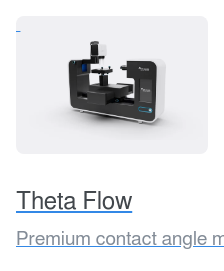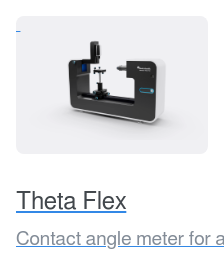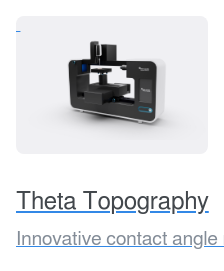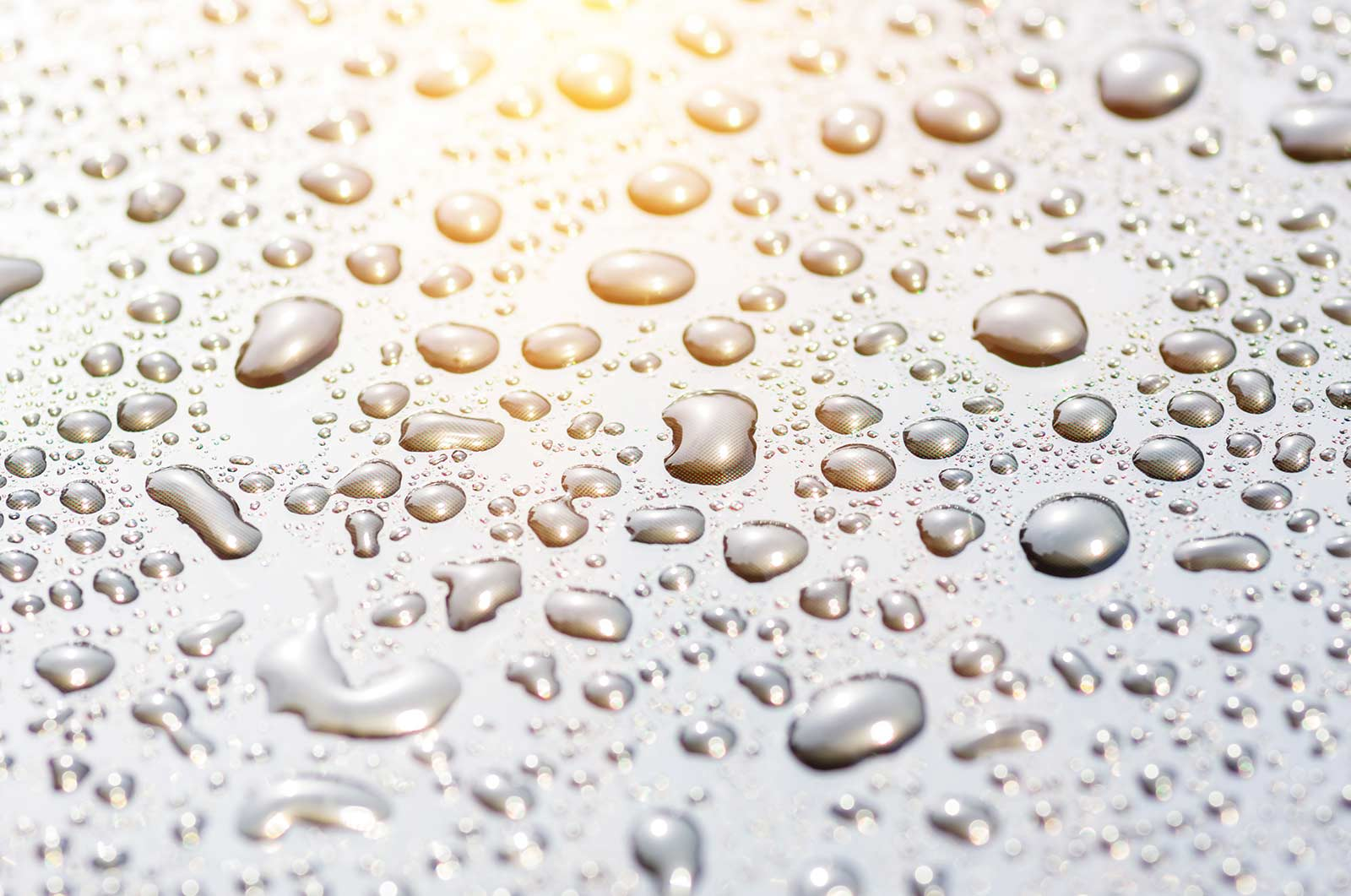# Surface Free Energy

Precise characterization of the solid material surface plays a vital role in research and product development in many industrial and academic areas. Surface free energy, or surface energy, is a property of the solid. Surface free energy is not accessible through direct measurement but instead, it is calculated by using the contact angle data. Surface free energy can be utilized to understand the interactions between the solid and liquid.

## What is surface free energy?

Surface free energy (SFE) can be considered as the surface tension of a solid. The most typical unit for SFE is mN/m, or its equivalent, dynes /cm. Surface free energy of the material arises from molecular interactions at the solid surface.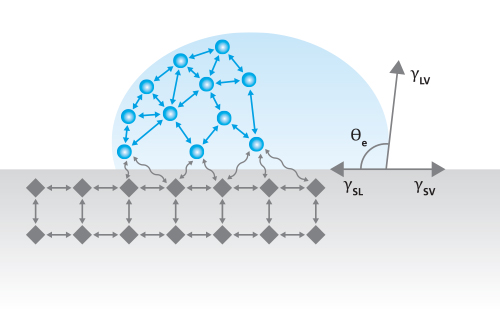The atoms at the surface don’t have the same number of neighboring atoms on their side which causes an excess force at the solid surface. The strength of this force is dependent on the strength of the forces between the atoms in the solid. For example, in metals, the atoms are linked together with extremely strong metal bonds. The surface free energy of the metals is thus typically very high. Then, on the other hand, bonds in polymeric materials are typically weak which makes the SFE of polymers low.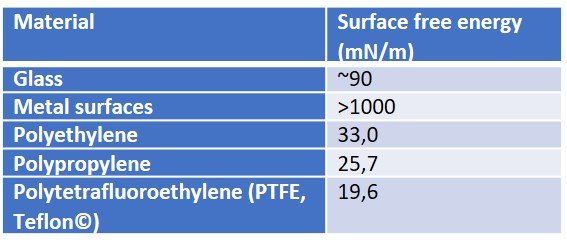Surface free energy determines how the solid behaves in contact with other materials. From a thermodynamic point of view, the high surface free energy materials are not stable and there is a need to minimize it. Many metals such as aluminum, will immediately when in contact with air (or another oxygen-containing atmosphere) grow the natural thin oxide layer on top to minimize the surface free energy.

## Surface free energy (SFE) theories

Most of the surface free energy theories have a basis in the understanding of the molecular interactions at the liquid-solid interface.

Young’s equation states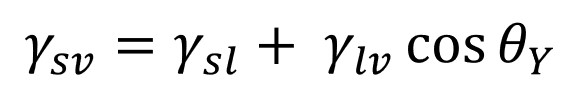, where γsv is the surface free energy, γsl is the interfacial tension between the liquid and the solid, γlv is the surface tension of the liquid, and θY is the contact angle on a surface. Out of these, the surface tension of the liquid and the contact angle can be easily measured. The interfacial tension between the solid and the liquid is the property that has caused a lot of debate during the last two centuries.

At the moment, the most used interfacial tension relationship is based on that presented by Girifalco and Good., where φ = 1 is assumed. This is the basis of the most used SFE theories still today.

### Owen, Wendt, Rabel and Kaelble (OWRK) method

OWRK is the most used surface free energy method. It separates the interactions at the liquid-solid interface into dispersive and polar. The idea that the quantity of the interfacial tension is determined by various interfacial interactions that depend on the properties of both the measuring liquid and the solid surface was presented by Fowkes. He assumed that the surface free energy of a solid and surface tension of a liquid is a sum of independent interactions such as dispersion, polar, hydrogen (related to hydrogen bonds), induction, and acid-base components. In OWRK method, the multicomponent approach is accepted but the interactions are divided into dispersive and polar, only. The polar interactions contain then all the other interactions (except the dispersive) mentioned above.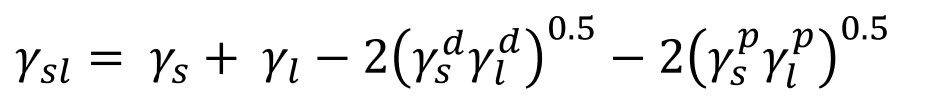### Wu method

Wu agreed with Owen et al. and divided surface free energy into dispersive and polar components. He, however, used a harmonic mean of the interfacial interactions instead of a geometric one. The interfacial tension could then be written as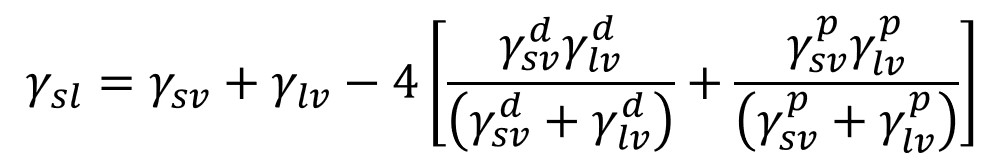### Van Oss - Chaudhury - Good (vOCG) theory

The newest theory was developed by Van Oss, Chaudhury, and Good. They divided the interactions into the long-range interactions which include London, Keesom, and Debye interactions. This component is called the Lifshitz-van der Waals component (γLW) and is equivalent to the dispersive part of the OWRK theory. The other part contains short-range interactions called the acid-base component (γAB) which is divided into acidic (γ+) and basic (γ-) constitutes.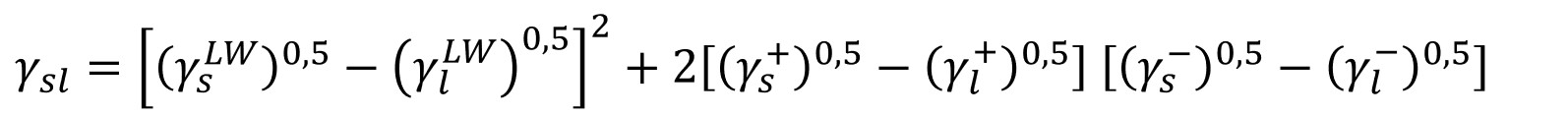This theory, sometimes also called acid-base theory is the second most used surface free energy theory. It has especially been utilized to look at interactions of proteins (and other biopolymers) with hydrophobic solids.## How to measure surface free energy in practice?

The most common way to calculate the surface free energy is through contact angle measurements. Pure liquids with the known surface tension are used for the measurements. Surface free energy is most typically measured through sessile drop measurements but the force tensiometer can be used as well.

### Surface free energy measurement with an optical tensiometer

The most common way to measure surface free energy is through sessile drop measurements with the optical tensiometer.

Depending on the surface free energy method utilized, two or three pure probe liquids are used for the measurement. Both OWRK and Wu contain two unknowns (the dispersive and polar parts of the solid SFE), so two liquids with the known surface tension components are needed to solve the equations. Acid-base theory (vOCG) has three unknown and thus three liquids are required when that is utilized.

The one liquid is polar and the other one should be completely dispersive. As a polar liquid, water is most typically used but ethylene glycol and glycerol are also possible. For the completely dispersive liquid, di-iodomethane or α-bromonaphthalene are chosen. This is because the two offer relatively high surface tension values despite being completely dispersive. High surface tension leads to measurable contact angles on most surfaces. Hydrocarbon such as hexane is not suitable due to very low surface tension of around 18 mN/m. In case, three liquids are used, the third one is typically ethylene glycol or some other polar liquid.

### Surface free energy measurement with force tensiometer

As force tensiometers can also be used to measure the contact angle of a solid surface, it is possible to use the contact angle data received with these measurements. However, one should note that as the force tensiometer is measuring the advancing and receding contact angles. For this reason, it is not possible to compare the surface free energy information obtained with different methods. It also varies which contact angle value is used for the calculations. Some use the advancing value while others calculate the average from the advancing and receding values.

One advantage of using the force tensiometer is that the surface free energy is an average value for the whole surface area being immersed into the measurement liquid.

Calculation of surface free energy of more unconventional samples such as thin fibers and powders is also is possible.

## Surface free energy on real surfaces

All the surface free energy theories rely on Young’s equation to be valid. Young’s equation, however, makes a lot of assumptions on the liquid, surface, and their interaction with each other. The purity of the liquid is relatively easy to guarantee but the solid surface should completely smooth and chemically homogeneous which is are both difficult requirements.

Especially the effect of surface roughness on the measured contact angle value has been discussed extensively as practically all surfaces are rough at some level. Wenzel equation gives the relation to the measured contact angle and the actual contact angle through the surface roughness factor. Combined contact angle and surface roughness measurements can be utilized to measure roughness corrected contact angles which are then used to calculate roughness corrected surface free energy values. With this method, the effect of roughness can be taken away from the surface free energy results. This is especially useful when different types of surface treatments and their effect on contact angle values are considered.

## Related products